# Disturbance Observer-assisted Trajectory Tracking Control for Surgical Robot Manipulator

Disturbance Observer-assisted Trajectory Tracking Control for Surgical Robot Manipulator
Department of Electrical and Electronics, University of Petroleum and Energy Studies, Dehradun 248006, India

Department of Analytics, University of Petroleum and Energy Studies, Dehradun 248006, India

Corresponding Author Email:
varnitaverma2@gmail.com
Page:
355-362
|
DOI:
https://doi.org/10.18280/jesa.520404
17 April 2019
|
Accepted:
14 July 2019
|
Published:
10 October 2019
| Citation

OPEN ACCESS

Abstract:

This paper attempts to control the motion of a surgical robot to perform surgeries in an accurate and precise manner. In the target robot, two revolute joints, one prismatic joint and one revolute joint are connected in series, i.e. the 2PRP configuration. The external disturbances on the 2PRP robot were estimated, and the dynamic modelling of the robot was performed using the Newton-Euler formulation. Then, a control system was designed to control the robot motion by the torque computed based on the data collected by the disturbance observer, which compensates for the disturbance-induced error. The surgical robot and control system were simulated in MATLAB/Simulink. The results show that the designed control system can effectively compensate for the position error and control the robot motion in surgeries.

Keywords:

nonlinear control, disturbance observer, kinematics, dynamic modeling, tracking

1. Introduction

The wide range of medical healthcare is equipped with robots in the current paradigm. The surgical robots play a crucial role in the healthcare domain. The increasing interest of robots in different surgical applications like orthopedic surgery, bariatric surgery, Cosmetic surgery, Ocular surgery, rehabilitation etc. [1-3]. The surgical manipulator is widely used in various surgical applications like cutting, suturing, catering, etc. The surgical manipulator is chosen specifically for suturing task and assumed to have in-vivo laceration [4, 5]. The configuration of the manipulator is 2RPR. It has horizontal jointed articulated arm configuration manipulator. The end tool of the robot can be modified to perform various surgical tasks such as a holding camera and smart tools like endo stitch, endosew, etc. .

As compared to the task performances of the surgical manipulator need to undergo various interactions between patient body parts and robot end tool. The robot will receive different interaction forces on interacting with different body parts due to the difference in stiffness coefficient. The interaction forces while interacting with the patient is assumed to be external disturbances represented by $\tau_{\text {extdis}}$. The internal disturbance of the manipulator represented by $\tau_{i n d i s}$ is considered to be caused by system uncertainty and noises. Therefore we need to design a controller who will have the knowledge of each state of the system and able to compensate for the effect of disturbances [7-9]. The common techniques used for disturbance rejection are such as Adaptive control, Robust Control, active Kalman filtering, Sliding mode control, etc. for the different robotic applications. The emerging technique used for disturbance compensation is known as disturbance observer. In the disturbance observer is popular because of considering the disturbance model acting on each joint of the robot and estimating the unknown parameters of the system such as torque and forces. The independent joint control the disturbance observer considers the varying load , dynamic uncertainties , friction force [12, 13], unmodeled dynamics as the lumped disturbance term . By the use of linear matrix inequality (LMI) the disturbance observer is designed for the slow varying disturbances. The SCARA robot configuration and Phantom Omni was taken into consideration for the validation of results . For the rapid time-varying disturbance like friction, the nonlinear disturbance observer technique was discussed by W. Chen  A Nonlinear disturbance observer using feedforward compensation technique without computing acceleration measurements which is useful for computing sensorless torque control and fault detection. Further Nikoobin et.al. modified Chen’s Method for the generalized nonlinear disturbance observer applicable for n DOF robot manipulator having revolute joints . Mohammadi et al.  provides nonlinear disturbance observer generalized solution for all serial manipulator irrespective of its joint configuration.

The scope of this research is to control the motion of the surgical robot in order perform the accurate and precise task of surgery. The joint errors in each joint of robot manipulator can cause a significant drift in the position of surgical tool. This drift in the surgical tool position can be the cause of huge loss such as death of patient. In order to compensate the error caused by disturbances, the disturbance observer-based computed torque control is used. The surgical robot is having 2RPR robot configuration.

Hence in this paper, the trajectory tracking of a surgical robot for the ex-vivo laceration. The robust and effective control scheme implement to compensate the disturbances is disturbance observer-based computed torque control. To estimate the states of the system when the measurements are not available the disturbance observer is the most effective technique for the same as proven by the literature [17, 19-24]. The Newton-Euler formulation is used for the dynamic modeling of the surgical robot [25, 26]. With the help of simulation modeling, the performance estimation of the defined surgical robot is been computed. The frame assignment of the manipulator is represented in Figure 1.

## 1.jpgFigure 1. Frame assignment of the surgical manipulator

The physical dimensions of the system along with mass parameters of the surgical manipulator is taken into consideration. To identify the dynamics and kinematics of the surgical manipulator. Therefore, Table 1 illustrates the parameters taken into consideration during the modeling of the manipulator in simulation.

Table 1. Surgical robot parameters and their value

For the kinematic and dynamic modeling of the surgical robot, the Denavit-Hartenberg (D-H) parameter of the robot is identified from the frame assignment diagram Figure 1. Table 2 represents the Denavit-Hartenberg (D-H) parameter of the surgical robot.

Table 2. D-H parameter of the surgical manipulator having RRPR configuration

 Joint Axis # Joint Angles $θ_i$ Joint twist di Link length ai Joint Offset $α_i$ 1 θ1 d1 l1 π 2 θ2 0 l2 0 3 0 d3 0 0 4 θ4 d4 0 0
2. Dynamic Modelling for Surgical Robot

There is n number of methods to formulate the dynamics of the system such as Euler Lagrange, Recursive Lagrange, Newton Euler, Bond Graph, Featherstone, Wittenberg algorithms, etc. [27, 28]. From which Newton Euler and Euler Lagrange are commonly used algorithms. Newton Euler is considered to be more efficient with respect to Euler Lagrange because less number of addition, multiplication, and athematic operations are used . Therefore, the computation of surgical manipulator dynamic equation the Newton Euler formulation is chosen. The generalized dynamic expression for N degree of freedom (DOF) robot manipulator with disturbances (τdis) can be seen in Eq. (1) [28-31].

$M(q) \ddot{q}+N(q, \dot{q}) \dot{q}+G(q)=\tau_{i / p}+\tau_{d i s}$        (1)

where, the Generalized Input Force Vector (n x 1 dimension) is expressed as $\boldsymbol{\tau}_{\boldsymbol{i} / \boldsymbol{p}}$, The Inertia Matrix of a surgical manipulator is represented by M with the dimension of n x n. M(q) is a positive symmetric matrix, N is Centrifugal and Coriolis Forces (n x 1 dimension), G is a Gravitational Force Vector, $\dot{q}$ is Joint Angular Velocity Vector and q is Joint Position Vector. τdis is stated as the total disturbance acting on each joint of the manipulator. The total disturbance is the algebraic sum of internal disturbances caused by model uncertainty and external disturbances caused by friction forces are stated in Eq. (2) .

$\tau_{\mathrm{dis}}=\tau_{\mathrm{indis}}+\tau_{\mathrm{extdis}}$      (2)

The internal and external disturbances in the system were modeled as mentioned in Eq. (3) and Eq. (4). 

$\tau_{\text {indis}}=\Delta M(q) \ddot{q}+\Delta N(q, \dot{q}) \dot{q}+\Delta G(q)+v$       (3)

where, $\Delta M(q) \ddot{q}, \Delta N(q, \dot{q}) \dot{q}$ and $\Delta G(q)$ are the representation of variation in system modeling from the actual system to the nominal system also known as parameter uncertainties. The system noises due to measurement and process are represented as v.

The modeling of external disturbances for the ith joint of the manipulator, i=1, 2, 3, 4 is stated below in Eq. (4). Where $\boldsymbol{f}_{r i}$ is the coefficient of friction, which includes coulomb, static and viscous friction. The parameters used for simulation are chosen as $f_{r 1}=1.43, f_{r 2}=1.6, f_{r 3}=1.4, f_{r 4}=1.2$ and t as a simulation time of the system.

$\tau_{\text {iextdis}}=f_{r i} * \operatorname{sgn}(2 * t+1)$        (4)

The Eq. (5) states the Inertia Matrix of the surgical robot .

$M(\mathrm{q})=\left[\begin{array}{cccc}{k 1+k 2 * \cos (\theta 2)} & {\mathrm{k} 3+0.5 * k 2 * \cos (\theta 2)} & {0} & {-k 5} \\ {k 3+0.5 * k 2 * \cos (\theta 2)} & {k 3} & {0} & {-k 5} \\ {0} & {0} & {k 4} & {0} \\ {-k 5} & {-k 5} & {0} & {k 5}\end{array}\right]$    (5)

The Coriolis Matrix $(N(q))$ is defined in Eq. (6).

$N(q)=\left[\begin{array}{cccc}{-k 2 * \sin (\theta 2) * \theta 2} & {-0.5 * k 2 * \sin (\theta 2) * \theta 2} & {0} & {0} \\ {0.5 * k 2 * \sin (\theta 2) * \theta 1} & {0} & {0} & {0} \\ {0} & {0} & {0} & {0} \\ {0} & {0} & {0} & {0}\end{array}\right]$    (6)

Eq. (7) states the Gravity Matrix (G) of the surgical robot, which shows the impact of gravity on each link of the manipulator.

$G(q)=\left[\begin{array}{c}{0} \\ {0} \\ {-k 4 * g} \\ {0}\end{array}\right]$     (7)

In order to simplify the dynamics of the manipulator, the arbitrary constants are used. The value of constants used is stated as constant $k 1=I1+I2+I3+I4+m 1 * l_{1}^{2}+S 1+S 2$. The Coriolis matrix is having Constant k2 which can be stated as $2 *\left(2 * l_{1} * l_{2} * m 2+4 * l_{1} * l_{2} *(m 3+m 4)\right)$. The constant k3 is stated as $I2+I3+I4+m 2 * l_{2}^{2}+4 * l_{2}^{2}(m 3+m 4)$ where k4 is the algebraic sum of m3 and  m4 and k5  is equal to the moment of Inertia of link 4 represented as I4. The other constant S1 and S2 stated in constant k1 were equivalent to $m 2\left(l_{2}^{2}+4 * l_{1}^{2}\right)$ and $(m 3+m 4) *\left(4 * l_{1}^{2}+4 * l_{2}^{2}\right)$ respectively.

Where the Moment of Inertia around the centroid is Ii, $m_{i}$ is the mass, $l_{i}$ is the length of link i. The Jacobian of the surgical manipulator is defined in Eq. (8), With respect to the robot base frame, is as follows:

$J(q)=\left[\begin{array}{cccc}{-l1 * \sin (\theta 1)-l2 * \sin (\theta 1+\theta 2)} & {-l2 * \cos (\theta 1+\theta 2)} & {0} & {0} \\ {l1 * \cos (\theta 1)+l2 * \cos (\theta 1+\theta 2)} & {l2 * \cos (\theta 1+\theta 2)} & {0} & {0} \\ {0} & {0} & {0} & {-1} & {0} \\ {1} & {1} & {0} & {1}\end{array}\right]$     (8)

3. Control Scheme of the Surgical Manipulator

The purpose of implementing the Disturbance Observer (DOB) control scheme on the surgical manipulator is to optimize the effect of both internal and external disturbances. From the literature reviewed, the system is assumed accurate and with the help of sensors external disturbances are measured [8, 28-31]. In the proposed surgical system, the measurement devices are considered though which the joint position of the manipulator is achieved.

## 2.jpg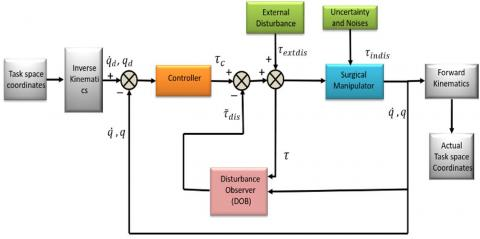Figure 2. Block Diagram of the disturbance observer scheme for the surgical robot

The desired task space trajectory is feed to the system through inverse kinematics such that the desired angle for each joint is computed. The error between desired and actual joint angles/ joint displacement is passed through control block diagram that provides the control torque represented as τc. Disturbance observer in order to compensate for the disturbance and achieve accurate trajectory tracking computed the estimates of disturbance stated in Eq. (10) .

The nonlinear control law for a surgical robot is stated in Eq. (9) .

$\tau_{i / p}=M(q)\left(\ddot{q}_{d}\right)+N(q, \dot{q}) \dot{q}+G(q)-\tilde{\tau}_{d i s}+k_{d} \dot{e}+k_{p} e$    (9)

On solving equation 1 with Eq. (9) we will get,

$M(q)\left[\ddot{e}+k_{d} \dot{e}+k_{p} e\right]=0$     (10)

Let's assume $\ddot{e}+k_{d} \dot{e}+k_{p} e=\tilde{\tau}_{d i s}$. From the above equation it states that $M(q) \tilde{\tau}_{d i s}=0$.The mass matrix of the system cannot be zero hence it states that $\tilde{\tau}_{d i s}=0$.

$\tau_{i / p}=\widehat{M}(q)\left(\ddot{q}_{d}+k_{d} \dot{e}+k_{p} e\right)+\widehat{N}(q, \dot{q}) \dot{q}+\hat{G}(q)-\tilde{\tau}_{d i s}$    (11)

where, the estimates of M(q) , $\tau_{d i s}$ , $N(q, \dot{q})$ and G(q) are represented as $\widehat{M}(q)$, $\tilde{\tau}_{d i s}$ , $\widehat{N}(q, \dot{q})$ and $\widehat{G}(q)$. The $\tilde{\boldsymbol{\tau}}_{d i s}$ is the represented as disturbance estimator with n x 1 matrix dimension. The $\tilde{\boldsymbol{\tau}}_{d i s}$ is equivalent to the product of the gain constant ($\boldsymbol{k}_{3}$) and joint velocity $(\dot{\boldsymbol{q}})$ with the algebraic sum of Z which is an arbitrary vector taken into consideration can be seen in the below Eq. (12).

$\tilde{\tau}_{d i s}=k_{3} \dot{q}+Z$      (12)

On taking the derivative of $\tilde{\boldsymbol{\tau}}_{d i s}$ stated in the above Eq. (12) will become as

$\dot{\tilde{\tau}}_{d i s}=k_{3} \ddot{q}+\dot{Z}$     (13)

On replacing $\ddot{\boldsymbol{q}}$ value from Eq. (13)

$\dot{\tilde{\tau}}_{d i s}=k_{3} M^{-1}\left(\tau+\tilde{\tau}_{d i s}-N-G\right)+\dot{Z}$      (14)

On rearranging the values of Eq. (14)

$\dot{Z}=-k_{3} M^{-1}\left(\tau+\tilde{\tau}_{d i s}-N-G\right)-\tilde{\tau}_{d i s}$      (15)

On replacing the value of $\tilde{\boldsymbol{\tau}}_{\boldsymbol{d} \boldsymbol{i} \boldsymbol{s}}$ from Eq. (15)

$\dot{Z}=-k_{3} M^{-1}\left(\tau+\tilde{\tau}_{d i s}-N-G\right)-k_{3} \dot{q}$       (16)

Eq. (16) is the final expression of the disturbance estimator of the proposed disturbance observer. The error causing in each joint of the manipulator due to internal and external disturbances provides the accumulative error at the end effector position. Similarly, to reduce the error in the position of surgical tool to locate the targeted position the error at each joint gets reduced using proposed disturbance observer control technique with successive operations. This observer requires only the measurement of joint velocity. The tuning of gain parameter of the DOB $k_{p}, k_{d},$ and $k_{3}$ brings the significant difference in system settling time and steady-state errors.

## 3.jpgFigure 3. Modelling of disturbance observer assisted surgical manipulator by MATLAB / Simulink

4. Simulation Study of Disturbance Observer

The mathematical modeling of the surgical manipulator and control system is implemented in Matlab/Simulink package shown in Figure 3. While modeling the system the external disturbances and internal disturbances were taken into consideration. The reference trajectory is the trajectory of laceration on the patient. The reference trajectory is given to the manipulator defined in Cartesian space. Through inverse kinematics, the joint space trajectory is feed to the system to achieve accurate and robust trajectory tracking. The results of the manipulator with and without disturbance observer are compared in presence of disturbance torque, as modeled in Eq. (1).

The gain values of the DOB control used during simulation are Kp = 180I, Kd= 36I, and K3= 10I.

## 4a.jpg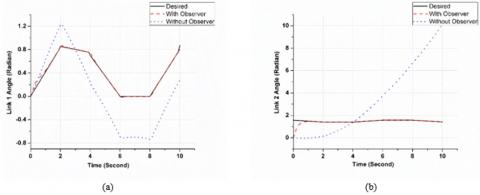## 4b.jpg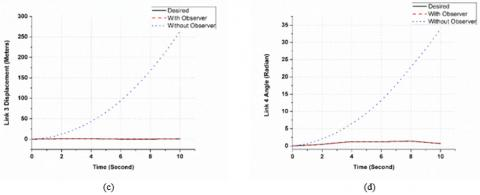Figure 4. Time trajectory position tracking of each link of the manipulator (a), (b), (c) and (d) with and without observer

## 5a.jpg## 5b.jpgIn Figure 4 the results of DOB, without DOB and desired trajectory of the joint angle of link1, link 2 and link 4 and length of displacement of link 3 in case of the prismatic joint is compared. The difference between the desired position and actual joint position is the position tracking error of the surgical manipulator while tracking laceration for suturing task. The position tracking error in with and without observer can be seen in Figure 5.

The joint disturbance tracking using disturbance observer in each joint of the surgical manipulator can be in Figure 6. This shows system would able to optimize the disturbance both internally and externally by tracing the required laceration trajectory. Figure 7 shows the error joint disturbance torque/force at each joint of the surgical manipulator for tracing the trajectory.

## 6a.jpg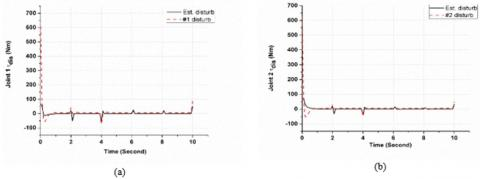## 6b.jpgFigure 6. Joint disturbance tracking profile for each joint of the surgical manipulator

## 7a.jpg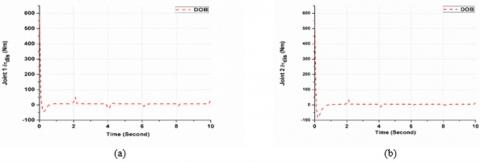## 7b.jpgFigure 7. Disturbance tracking error in each joint of a surgical manipulator

In the task-space coordinates, the performance of DOB controller with respect to without DOB controller can be seen in Figure 8. The trajectory of laceration on the patient is tracked effectively and adapting disturbances by disturbance observer control. This task of surgical robot considered in the system is to track the desired trajectory and compensate for the disturbance caused by internal and external factors in order to achieve precise motion of manipulator for suturing task.

## 8.jpg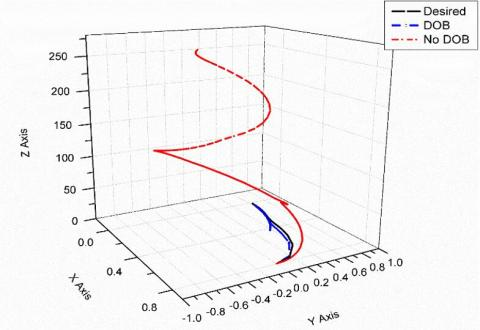Figure 8. Task space coordinate (XYZ) of the end effector during laceration tracking operation

The performance estimation of the controller can be through the root mean square (RMS) error for the position and disturbance tracking. The below mentioned Eq. (13) and Eq. (14) is used for estimation of RMS error.

$q_{\text {rms}_{-} \text {error}}=\sqrt{\sum_{i=1}^{n} \frac{\left(q_{d}-q_{i}\right)^{2}}{n}}$       (13)

$\tau_{d i s_{-} r m s_{-} e r r o r}=\sqrt{\sum_{i=1}^{n} \frac{\left(\tau_{d}-\tau_{i}\right)^{2}}{n}}$         (14)

Table 3. Illustrate the RMS value of position tracking error and disturbance tracking error while performing the surgical task by the manipulator

 RMS error Joint 1 Joint 2 Joint 3 Joint 4 Position Tracking 0.06902 0.01774 0.05902 0.007978 Disturbance Tracking 5.559 3.355 1.403 1.197

It is observed that uncertainties, external disturbances and dynamic changes in the system are well adapted by the disturbance observer control. The simulation results are intuitive and point out the effectiveness and use of control for a surgical robot. The laceration tracking performance of controller extended the work to an autonomous surgical robot. The accurate and robust tracking performance of control enables the surgical robot to do n number of tasks performed during surgery.

5. Conclusion

This paper presented the nonlinear disturbance observer to stabilized trajectory tracking for surgical robotic application. The application of this scheme is to test the 2RPR surgical robotic manipulator with the end tool as a suturing tool. The mathematic modeling of surgical robotic manipulator was discussed. The computation of external disturbances affecting the system for the surgical task. The laceration tracking operation was done successfully in order to assist the surgeon to perform suturing task. The obtained results illustrate the feasibility of the DOB control in the application surgical task.

Acknowledgment

The SEED Grant of Research & Development and Center of excellence in University of Petroleum and Energy Studies, Dehradun support this work.

References

 Piltan, F., Taghizadegan, A., Sulaiman, N.B. (2015). Modeling and control of four degrees of freedom surgical robot manipulator using MATLAB/SIMULINK. Int. J. Hybrid Inf. Technol., 8(11): 4778.

 Guess, T.M., Maletsky, L.P. (2005). Computational modelling of a total knee prosthetic loaded in a dynamic knee simulator. Med. Eng. Phys., 27(5): 357-367. https://doi.org/10.1016/j.medengphy.2004.11.003

 Gupta, A., Mondal, A.K., Gupta, M.K. (2019). Kinematic, dynamic analysis and control of 3 DOF upper-limb robotic exoskeleton. J. Eur. des Systèmes Autom., 52(3): 297-304. https://doi.org/10.18280/jesa.520311

 Shademan, A., Decker, R.S., Opfermann, J.D., Leonard, S., Krieger, A., Kim, P.C. (2016). Supervised autonomous robotic soft tissue surgery. Sci. Transl. Med., 8(337). http://dx.doi.org/10.1126/scitranslmed.aad9398

 Shademan, A., Decker, R.S., Opfermann, J.D., Leonard, S., Krieger, A., Kim, P.C.W. (2016). Supervised autonomous robotic soft tissue surgery. Science Translational Medicine, 8(337): 337ra64. https://doi.org/10.1126/scitranslmed.aad9398

 Verma, V., Chowdary, V., Gupta, M.K., Mondal, A.K. (2018). IoT and robotics in Healthcare. In Medical Big Data and Internet of Medical Things, Boca Raton : Taylor & Francis, : CRC Press, 2018, pp. 245-269. https://doi.org/10.1201/9781351030380

 Sariyildiz, E., Oboe, R., Ohnishi, K. (2019). Disturbance observer-based robust control and its applications: 35th anniversary overview. IEEE Trans. Ind. Electron. https://doi.org/10.1109/TIE.2019.2903752

 Hao, S., Hu, L., Liu, P.X. (2019). Sliding mode control for a surgical teleoperation system via a disturbance observer. IEEE Access, 7: 43383-43393. https://doi.org/10.1109/ACCESS.2019.2901899

 Anang, N.A., Abdullah, L., Jamaludin, Z., Chiew, T.H., Maharof, M., Salim, S.N.S., Retas, Z., Junoh, S.C.K. (2017). Tracking performance of NPID controller for cutting force disturbance of ball screw drive. J. Mech. Eng. Sci., 11(4): 3227-3239. https://doi.org/10.15282/jmes.11.4.2017.25.0291

 Pillai, B.M., Suthakorn, J. (2019). Motion control applications : Observer based DC motor parameters estimation for novices. Int. J. Power Electron. Drive Syst., 10(1): 195-210. http://doi.org/10.11591/ijpeds.v10.i1.pp195-210

 Singh, Y., Santhakumar, M. (2015). Inverse dynamics and robust sliding mode control of a planar parallel (2-PRP and 1-PPR) robot augmented with a nonlinear disturbance observer. Mech. Mach. Theory, 92: 29-50. https://doi.org/10.1016/j.mechmachtheory.2015.05.002

 Huang, S., Liang, W., Tan, K.K. (2019). Intelligent friction compensation : A review. IEEE/ASME Trans. Mechatronics, 24(4): 1763-1774. https://doi.org/10.1109/TMECH.2019.2916665

 Do, T.N., Tjahjowidodo, T., Lau, M.W.S., Phee, S.J. (2015). Nonlinear friction modelling and compensation control of hysteresis phenomena for a pair of tendon-sheath actuated surgical robots. Mech. Syst. Signal Process., 1-15. https://doi.org/10.1016/j.ymssp.2015.01.001

 Chen, W.H., Yang, J., Guo, L., Li, S. (2016). Disturbance-observer-based control and related methods - an overview. IEEE Trans. Ind. Electron., 63(2): 1083-1095. https://doi.org/10.1109/TIE.2015.2478397

 Mohammadi, A., Marquez, H.J., Tavakoli, M. (2011). Disturbance observer-based trajectory following control of nonlinear robotic manipulators. Proc. 23rd Can. Congr. Appl. Mech., pp. 779-782.

 Nikoobin, A., Haghighi, R. (2009). Lyapunov-based nonlinear disturbance observer for serial n-Link robot manipulators. J. Intell. Robot. Syst. Theory Appl., 55(2-3): 135-153. https://doi.org/10.1007/s10846-008-9298-2

 Mohammadi, A., Tavakoli, M., Marquez, H.J., Hashemzadeh, F. (2013). Nonlinear disturbance observer design for robotic manipulators. Control Eng. Pract., 21(3): 253-267. https://doi.org/10.1016/j.conengprac.2012.10.008

 Liu, X., Zuo, G., Zhang, J., Wang, J. (2019). Sensorless force estimation of end-effect upper limb rehabilitation robot system with friction compensation. Int. J. Adv. Robot. Syst., 1219: 1-11. https://doi.org/10.1177/1729881419856132

 Santhakumar, M. (2013). A nonregressor nonlinear disturbance observer-based adaptive control scheme for an underwater manipulator. Adv. Robot., 27(16): 1273-1283. https://doi.org/10.1080/01691864.2013.819608

 Ding, S., Chen, W.H., Mei, K., Murray-smith, D. (2019). Disturbance observer design for nonlinear systems represented by input-output models. IEEE Transactions on Industrial Electronics, 67(2): 1222-1232. https://doi.org/10.1109/TIE.2019.2898585

 Habibollahi, A., Nikoobin, A., Dideban, A. (2018). Lyapunov-based nonlinear disturbance observer for n-link flexible joint robot manipulators. Control Eng. Appl. INFORMATICS, 20(2): 22-32. https://doi.org/10.1007/s10846-008-9298-2

 Homayounzade, M., Khademhosseini, A. (2019). Disturbance observer-based trajectory following control of robot manipulators. Int. J. Control. Autom. Syst., 17(1): 203-211. http://dx.doi.org/10.1007/s12555-017-0544-x

 Chen, W.H., Ballance, D.J., Gawthrop, P.J., O’Reilly, J. (2000). A nonlinear disturbance observer for robotic manipulators. IEEE Trans. Ind. Electron., 47(4): 932-938. http://doi.org/10.1109/41.857974

 Agarwal, V., Parthasarathy, H. (2019). Simultaneous estimation of parameter uncertainties and disturbance trajectory for robotic manipulator. Sadhana - Acad. Proc. Eng. Sci., 44(5). https://doi.org/10.1007/s12046-019-1092-2

 Djuric, A., Al Saidi, R., Elmaraghy, W. (2012). Dynamics solution of n -DOF global machinery model. Robot. Comput. Integr. Manuf., 28(5): 621-630.

 Hollerbach, J.M. (1980). A recursive lagrangian formulation of maniputator dynamics and a comparative study of dynamics formulation complexity. IEEE Transactions on Systems, Man, and Cybernetics, 10(11): 730-736. https://doi.org/10.1109/TSMC.1980.4308393

 Issa, A., Aqel, M.O.A., Tubail, A., Alkayyali, Y., Alay, A., Ferwana, M. (2017). Vision assisted SCARA manipulator design and control using arduino and LabVIEW. 2017 International Conference on Promising Electronic Technologies (ICPET), Deir El-Balah, Palestine. https://doi.org/10.1109/ICPET.2017.16

 Meera, C.S, Mukul Kumar, G., Santhakumar, M. (2019). Disturbance observer-assisted hybrid control for autonomous manipulation in a robotic backhao. Archive of Mechanical Engineering, 66(2): 153-169. https://doi.org/10.24425/ame.2019.128442

 Venkatesan, V., Mohan, S., Kim, J. (2014). Disturbance observer based terminal sliding mode control of an underwater manipulator. In 2014 13th International Conference on Control Automation Robotics & Vision (ICARCV), pp. 1566-1572. https://doi.org/10.1109/ICARCV.2014.7064549

 Soltanpour, M.R., Shafiei, S.E. (2010). Robust adaptive control of manipulators in the task space by dynamical partitioning approach. Elektron. ir Elektrotechnika, 101(5): 73-78.

 Cao, P., Gan, Y., Dai, X. (2019). Finite-time disturbance observer for robotic manipulators. Sensors (Basel)., 19(8): 1-11. https://doi.org/10.3390/s19081943